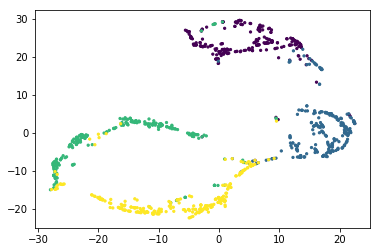# Getting started with Fermat¶

This notebook introduces the basic concepts in order to compute Fermat distance using the Fermat package.

We use a toy data set (the swiss roll) in order to illustrate.

Contents:

o Data generation
o Computing Fermat distance
o Visualization using t-sne
In :
import numpy as np
from scipy.spatial import  distance_matrix
from sklearn.manifold import TSNE

from mpl_toolkits.mplot3d import Axes3D
import matplotlib.pyplot as plt

from generate_data import generate_swiss_roll


If you prefer to work without installing Fermat package, then you can import directly the files from the fermat folder

In :
#import sys
#path_to_FermatFolder = '/home/facu/Dropbox/Repositorios/Fermat-distance'
#sys.path.append(path_to_FermatFolder)

In :
from fermat import Fermat

In :
help(Fermat)

Help on class Fermat in module fermat.Fermat:

class Fermat(builtins.object)
|  Methods defined here:
|
|  __init__(self, alpha, path_method='L', k=None, landmarks=None, estimator='up', seed=None)
|      Initialization of the Fermat model
|
|      Parameters
|      -----------
|      alpha: float
|          Parameter of the Fermat distance.
|
|      path_method: string ['FW','D','L']
|
|          Options are:
|
|                  'FW'    -- Computes the exact Fermat distance using the Floyd-Warshall algorithm. The complexity is
|                           O[N^3] where N is the number of data points.
|
|                  'D'     --  Computes an approximation of the Fermat distance using k nearest neighbours and the
|                           Dijkstra algorithm. The complexity is O[N*(k*N*log N)]
|
|                  'L'     -- Computes an approximation of the Fermat distance using landmarks and k-nn. The complexity
|                           is O[l*(k*N*log N)] where l is the number of landmarks considered.
|
|      k: integer, optional
|          Number of nearest neighbors to be considered.
|          Incompatible with path_method == 'FW'
|
|      landmarks: integer, optional
|          Number of landmarks considered in the Fermat distance computation.
|          Only available when path_method = 'L'
|
|      estimator: string ['up', 'down', 'mean', 'no_lca'] (default: 'up')
|          When computing an approximation of the Fermat distance, there are lower and upper bounds of the true value.
|          If estimator == 'no_lca', the distance for a pair of points is calculated as the minimum sum of the distance
|              from both points to one of the landmarks.
|          If estimator == 'up', the distance for a pair of points is calculated as the minimum sum of the distance
|              from both points to the lowest common ancestor in the distance tree of one of the landmarks.
|          If estimator == 'down', the distance for a pair of points is calculated as the maximum difference of the
|              distance from both points to one of the landmarks.
|          If estimator == 'mean', the  mean between 'up' and 'down' estimators.
|          Only available when path_method = 'L'
|
|      seed: int, optional
|          Only available when path_method = 'L'
|
|
|      Returns
|      -----------
|      Fermat class object
|
|
|      Examples
|      -----------
|      # init an exact Fermat distance model
|      f_exact = Fermat(alpha = 3, path_method='FW', seed = 1)
|
|      # init an approx Fermat distance model
|      f_aprox = Fermat(alpha, path_method='L', k=10, landmarks=50)
|
|  fit(self, distances)
|      Parameters
|      -----------
|      distances: np.matrix
|          Matrix with pairwise distances
|
|  get_distance(self, a, b)
|      Parameters
|      -----------
|      a: int
|          Index of a data point
|
|      b: int
|          Index of a data point
|
|      Returns
|      -----------
|      Float: the Fermat distance between points a and b
|
|  get_distances(self)
|      Parameters
|      -----------
|      -
|
|      Returns
|      -----------
|      np.matrix with the pairwise Fermat distances
|
|  ----------------------------------------------------------------------
|  Data descriptors defined here:
|
|  __dict__
|      dictionary for instance variables (if defined)
|
|  __weakref__
|      list of weak references to the object (if defined)



## Data generation¶

Generate the Swiss Roll data set.

In :
data, labels = generate_swiss_roll(oscilations = 15, A = 3, n = 250)
print('Data dimension:{}'.format(data.shape))

Data dimension:(1000, 3)


Visualize the data

In :
fig = plt.figure()

ax.view_init(10, 80)
ax.scatter(xs=data[:,0], ys=data[:,1], zs=data[:,2], c=labels, s=4)
plt.title('Swiss Roll Normals dataset \n N=%s'%(data.shape))

plt.show()Compute euclidean distances between points in the data set

In :
distances = distance_matrix(data,data)


## Computing Fermat-Distances¶

Parameters

In :
alpha = 3

k = 100
landmarks = 30


#### Exact method: computes all the pairwise Fermat distances in an exact way¶

In :
%%time

# Initialize the model
f_exact = Fermat(alpha = alpha, path_method='FW')

# Fit
f_exact.fit(np.matrix(distances))

CPU times: user 1.04 s, sys: 12.1 ms, total: 1.05 s
Wall time: 1.05 s

In :
fermat_dist_exact = f_exact.get_distances()


#### Aprox method 1: using k-nearest neighbours¶

In :
%%time

# Initialize Fermat model
f_aprox_D = Fermat(alpha, path_method='D', k=k)

# Fit
f_aprox_D.fit(np.matrix(distances))

CPU times: user 1.46 s, sys: 0 ns, total: 1.46 s
Wall time: 1.46 s

In :
fermat_dist_aprox_D = f_aprox_D.get_distances()


#### Aprox method 2: using landmarks and k-nearest neighbours¶

In :
%%time

# Initialize Fermat model
f_aprox_L = Fermat(alpha, path_method='L', k=k, landmarks=landmarks)

# Fit
f_aprox_L.fit(np.matrix(distances))

CPU times: user 513 ms, sys: 2.88 ms, total: 516 ms
Wall time: 524 ms

In :
fermat_dist_aprox_L = f_aprox_L.get_distances()


## Visualization¶

Visualization for the Fermat distances using t-SNE

In :
tsne_model = TSNE(n_components=2, verbose=0, perplexity=50, n_iter=500)
tsnes = tsne_model.fit_transform(fermat_dist_exact)

In :
plt.scatter(tsnes[:,0],tsnes[:,1], c = labels, s = 5)

Out:
<matplotlib.collections.PathCollection at 0x7f864feb74e0>